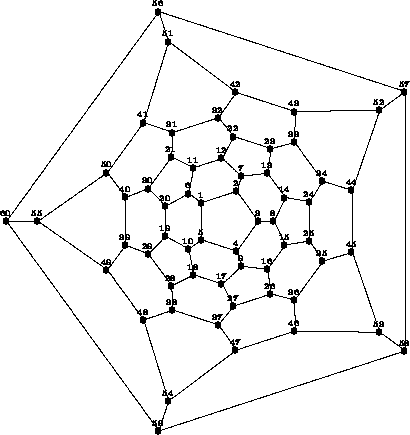The action on the set of vertices

## The action on the set of verticesLabelling the vertices of the fullerene C60, given by its Schlegel diagram

Labelling the vertices of the fullerene C60 as is indicated in figure the permutation representation of generators of R and S acting on the set of vertices is given by:

p1= (56,57,58,59,60)(51,52,53,54,55)(42,44,46,48,50)(41,43,45,47,49)(32,34,36,38,40) (31,33,35,37,39)(22,24,26,28,30)(21,23,25,27,29)(12,14,16,18,20)(11,13,15,17,19) (6,7,8,9,10)(1,2,3,4,5)

p2= (37,38,48,54,47)(27,28,49,59,46)(18,39,60,53,26)(17,29,55,58,36)(10,40,56,45,16) (9,19,50,57,35)(7,12,22,23,13)(5,30,51,44,15)(4,20,41,52,25)(3,6,31,43,24) (2,11,32,33,14)(1,21,42,34,8)

s= (58)(57,59)(56,60)(53)(52,54)(51,55)(45,46)(44,47)(43,48)(42,49)(41,50)(35,36) (34,37)(33,38)(32,39)(31,40)(25,26)(24,27)(23,28)(22,29)(21,30)(15,16)(14,17)(13,18) (12,19)(11,20)(8,9)(7,10)(6)(3,4)(2,5)(1)

p1 is a 5-fold rotation around the centre of figure and p2 is a 5-fold rotation, where the rotation axis goes through the centres of the faces {7,12,22,23,13} and {37,38,48,54,47} .

There are computer algebra systems which evaluate the cycle index of a permutation group given by a set of generators. For instance the SYMMETRICA routine `zykelind_arb(a,b)` computes the cycle index `b` of a permutation group with generators given in the `VECTOR`-object `a`. In this way the cycle indices of R and S acting on the set of vertices are computed as

Z(R,Vertices)=(1)/(60)(24 x512 +20 x320 +15 x230 +x160)
Z(S,Vertices)=(1)/(120)(24 x106 +20 x610 +24 x512 +20 x320 +16 x230 +15 x14 x228 +x160).

harald.fripertinger@kfunigraz.ac.at,
last changed: January 23, 2001The action on the set of vertices